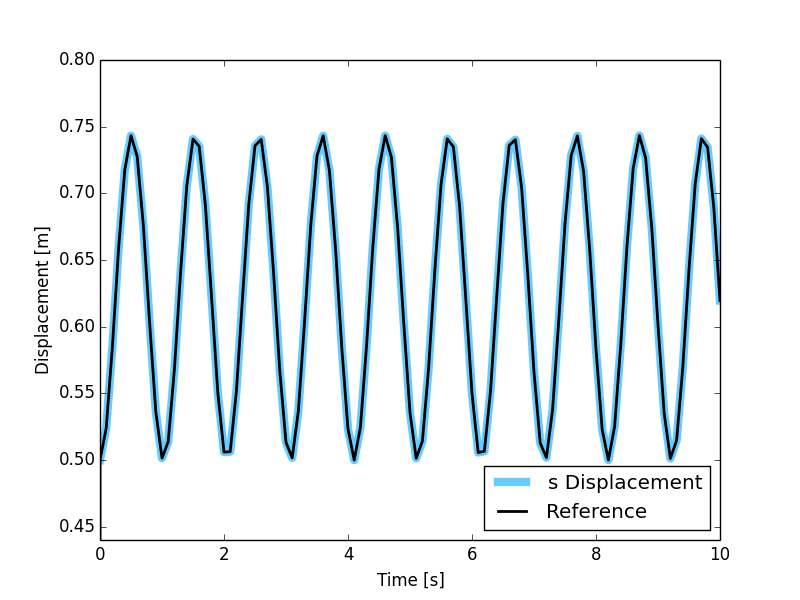# MBS Benchmark A05: Stiff Flyball Governor¶

## Benchmark Objective¶

The A05 MBS benchmark problem is an example of a stiff mechanical system.

## Benchmark Description¶

The A05 benchmark problem is also known as stiff flyball governor and was invented by J. Watt in the 18th century.

In this stiff mechanical system, coupler rods are substituted by spring-damper elements.

Shaft, rods and slider can be modeled as prismatic bodies with the characteristics provided in the next table. Two 5 kilograms point mass are placed on points colored in purple in the previous figure.

System Properties and Configuration
Shaft, Rods dimensions $$1.0\times0.01\times0.01 m$$
Slider dimensions $$0.1\times0.1\times0.1 m$$
Density $$\rho$$ $$3000 kg/m^3$$
Point masses 5 kg
Spring stiffness $$K$$ 8e5 N/m
Spring damping $$C$$ 4e4 Ns/m
Spring rest length 0.5 m
:$$s$$ 0.5 m
:$$\alpha$$ 30 degree
:$$\dot{\omega}$$ $$2\pi rad/s$$

## Results¶

The dynamic simulation of the A05 benchmark was executed for 10 s. The starting position of the system in shown in the previous figure and numerical values are reported in the previous table.

$$s$$ values estimated with the OpenSim simulation are compared with the values provided as reference [GDLC06].Figure 15: Comparison between OpenSim simulation results for coordinate $$s$$ (colored line) and MBS benchmark reference value (black dashed line).

### Computational Performances¶

To compute the simulation the developed implementation required 0.233 seconds.

Results obtained using an Intel Core i5-4570 @ 3.2 GHz computer running Windows 8.1 64-bit. OpenSim official release used: 3.2.

Comparision with other dynamic engines has been conducted submitting the obtained results to the benchmark collaborative library. Click here to see the comparison results.